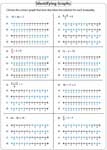Two-Step Inequalities Worksheets

This page contains a lot of two-step inequalities worksheets based on solving and graphing. Easy level has positive integer coefficients with answers only in positive numbers. Moderate level contains positive coefficients, with variables on any sides and answers in integers. Difficult level contains both positive and negative coefficients. This is applicable only for either solving or graphing or both.

Translating to Algebraic Expressions

Two-step inequalities are expressed in verbal phrases. Translate each phrase into an algebraic expression.Easy:

Moderate:

Identifying solutions

There are four choices given for each two-step inequality. Circle the solutions that satisfy each inequality.Solving Two-Step Inequalities

Each worksheet has 12 problems solving two-step inequalities.Easy:

Moderate:

Difficult:

Fractions:

Decimals:

Solutions in Interval Notation

For each two-step inequality, four choices are given in the interval notation. Identify the solutions that best suits each problem.Easy:

Moderate:

Solving and Graphing Two-Step Inequalities

Each worksheet has two skills, solving two-step inequalities and graphing the solutions on the number lines.Easy:

Moderate:

Difficult:

Fractions:

Decimals:

Identifying Graphs

Identify the correct solution graph for each two-step inequality.Easy:

Moderate:

Identifying Two-step Inequality

Solve each inequality from the choices and identify the one that has the solution graph.Easy:

Moderate:

Stock up your library with the complete set of worksheets on two-step inequalities.

Related Worksheets